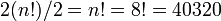# Double cover of alternating group:A8

View a complete list of particular groups (this is a very huge list!)[SHOW MORE]

## Definition

The double cover of alternating group:A8, denoted$2 \cdot A_8$, is defined as the double cover of alternating group for alternating group:A8. It is the unique Schur covering group for alternating group:A8, whose Schur multiplier is cyclic group:Z2.

## Arithmetic functions

Want to compare and contrast arithmetic function values with other groups of the same order? Check out groups of order 40320#Arithmetic functions
Function Value Similar groups Explanation for function value
order (number of elements, equivalently, cardinality or size of underlying set) 40320 groups with same order As$2 \cdot A_n, n = 8$:$2(n!)/2 = n! = 8! = 40320$

## GAP implementation

Description Functions used
PerfectGroup(40320,3) PerfectGroup
SchurCover(AlternatingGroup(8)) SchurCover, AlternatingGroup